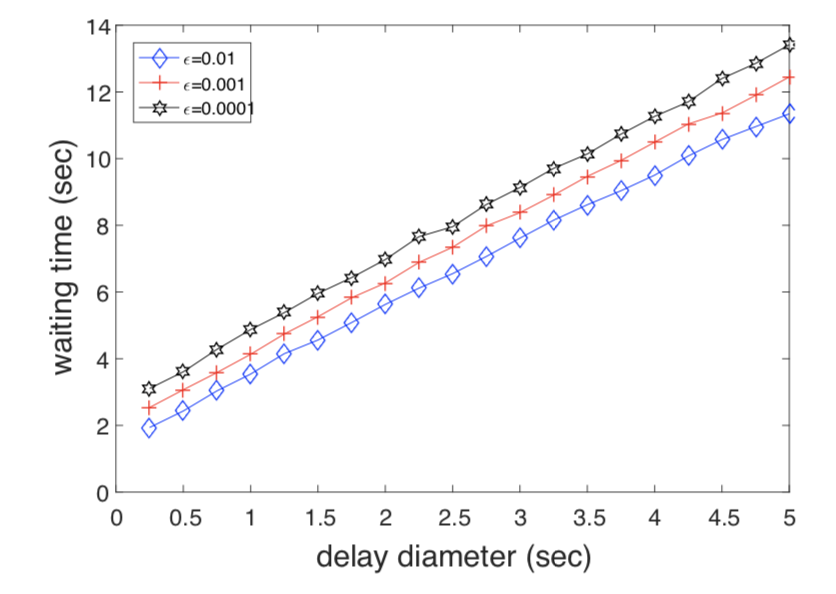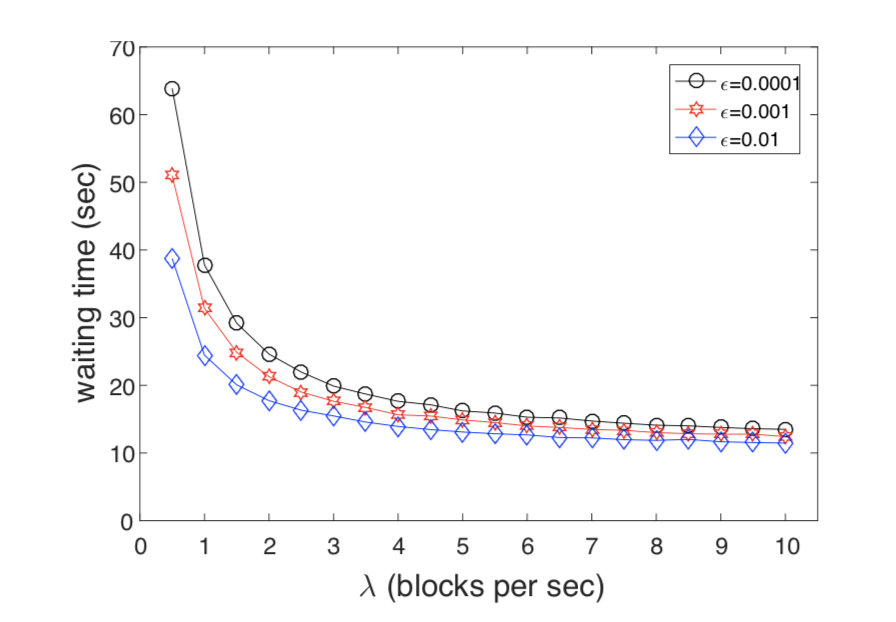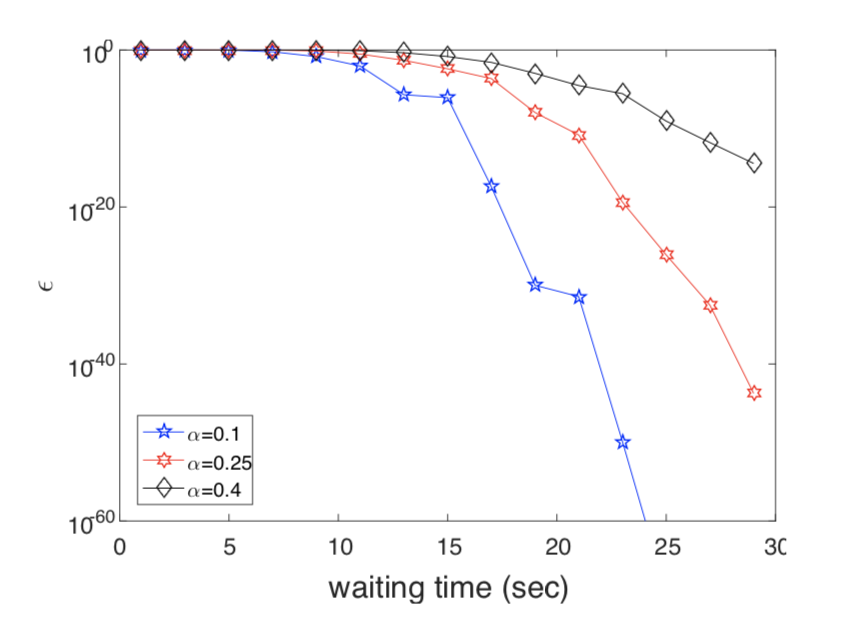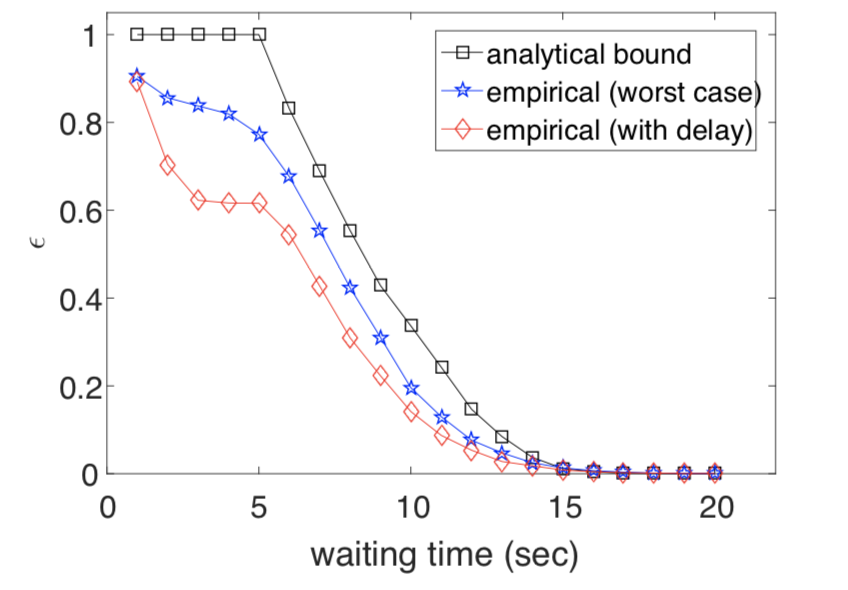Scroll

Categories

Tags

Monthly Archives

Keyword

Back

2018.07.26

# 附录B 模拟结果

We implemented the SPECTRE protocol in Python along with an event-driven simulator of network dynamics. For each experiment we generated an Erd˝os-R´enyi random network topology with 20 nodes. Each node forms 5 outgoing links, in expectation. The delay on each link was uniformly distributed and later scaled linearly so that the diameter of the graph is D (for the given D). Every point represents the average outcome over at least 500 experiments.

The main beneﬁt of SPECTRE is fast transaction conﬁrmation. The asymptotic waiting times derived from our formal analysis are in $O(\frac{ln(1/\varepsilon )}{\lambda (1-2\alpha )}+\frac{D}{1-2\alpha })$. In order to measure the actual waiting times, we utilized the online acceptance policy derived by Alg. 7. Accordingly, we stress that the merchant needs to wait additional D seconds in order to verify that no double-spend has been released in the past D seconds, as explained at the end of Appendix C. 18

SPECTRE的主要好处是快速的交易确认。 通过我们的形式化分析得到渐进等待时间在 $O(\frac{ln(1/\varepsilon )}{\lambda (1-2\alpha )}+\frac{D}{1-2\alpha })$ 以内。 为了测量实际的等待时间，我们采用了算法7中生成的在线接受策略。 因此，我们强调商家需要额外等待D秒才能确认在过去的D秒内没有发生双花，如附录C末尾所述。

How does the delay diameter affect acceptance times? Given that block creation rate is high, most of the waiting time for acceptance is dominated by the block propagation delay. Fig. 5 depicts the transaction acceptance times of SPECTRE, for various values of the delay diameter D, and for different security thresholds. Note that, unlike the Nakamoto Consensus, D affects the acceptance time of transactions but not their security.Fig. 5: The average time for a transaction to enter RobustTxO, assuming there’s no visible double-spend, for $\lambda = 10$ blocks per second and $\alpha = 0.25$.

How does the block creation rate affect acceptance times? Fig. 6 depicts the acceptance times for various values of the block creation rate $\lambda$, under a constant delay $d = 5$ seconds. The graph reafﬁrms the role of $\lambda$ in our asymptotic bound: accelerating the block creation process allows for faster acceptance times. For comparison, Bitcoin’s block creation rate of 1/600 implies waiting times that are orders of magnitudes higher (not plotted).

Fig. 6: The average time for a transaction to enter RobustTxO, assuming there’s no visible double-spend, for d = 5 seconds and $\alpha = 0.25$.

Can an attacker delay acceptance? We now turn to demonstrate the effect of censorship attacks in which some dishonest nodes publish blocks that do not reference other miners’ blocks. Recall that the Weak Liveness property of SPECTRE (Proposition 3) guarantees fast acceptance of transactions that are not visibly double-spent, even in the presence of a censorship attack. However, such an attack still causes some delay in transaction acceptance, but this delay is minor for small attackers.

In Fig. 7 we quantify this effect, by comparing the acceptance times in “peace days” to those under an active censorship attack. The parameters here are d = 5 seconds, $\lambda = 10$ blocks per second, and $\varepsilon = 0.01$. The results display a modest effect of the attack, and they show that in order to delay transaction acceptance by more than 5 to 10 seconds an attacker must possess a signiﬁcant share of the computational power in the network.Fig. 7: The average time for a transaction to enter RobustTxO, assuming there’s no visible double-spend, for $d = 5$ seconds, $\lambda = 10$ blocks per second, and $\varepsilon = 0.01$, in the presence and in the absence of a censorship attack.

How does $\varepsilon$ decrease for various sizes of the attacker? Once an honest node $\varepsilon$-accepts a transaction, there’s still a small risk ($\varepsilon$) that it would eventually be rejected. We show that the probability of this event vanishes quickly, even for an extremely capable attacker (e.g., with $\alpha = 0.4$ of the hashrate). This is illustrated in Fig. 8, assuming $d = 5$ seconds and $\lambda = 10$ blocks per second (notice that the y-axis is in log scale).

How tight is our security analysis? The analysis on which Alg. 3 relies makes several worst-case assumptions in order to bound the probability of a successful attack, e.g., that the attacker can broadcast blocks to and receive blocks from all nodes without any delay (see Appendix E, mainly Lemmas 14 and 20).

Accordingly, the analysis is not tight, and in reality attacks are in fact less likely to succeed. In Fig. 9, we depict the comparison between the analytical bound and two different empirical simulations. In these simulations we explicitly generate blocks for the attacker and simulate the optimal double-spending attack. We repeat the experiment 10,000 times for each point in the graph, and measure the empirical success rate.

The simulations assume two types of attackers: a worst-case attacker that is able to transmit and receive blocks with no delays, and a more realistic attacker that is connected to other nodes with typical delays. We compared the fraction of successful attacks under these setups to the analytical risk calculated by SPECTRE’s policy (Alg. 7).

The results show that the risk considered by SPECTRE’s RiskTxAccept indeed upper bounds the actual risk, and that transactions are even safer than we guarantee formally.Fig. 8: The probability of a successful double-spending attack, as a function of the waiting time before acceptance, under $d = 5$ seconds and $\lambda = 10$ blocks per second, for $\alpha$ = 0.1, 0.25, and 0.4. The probability here is the result of the calculation performed by Alg.3.Fig. 9: The analytical vs. empirical probabilities of a successful double-spending attack, as a function of the waiting time before acceptance, under $d = 5$ seconds, $\lambda = 10$, and $\alpha = 0.25$.

Top